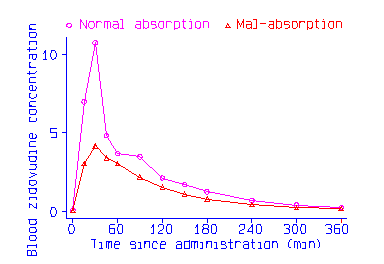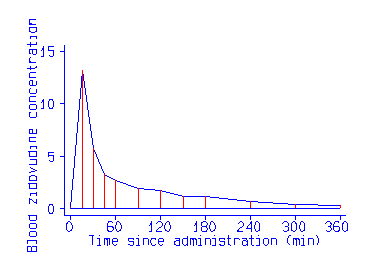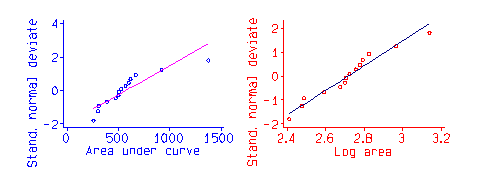#### Extract from "An Introduction to Medical Statistics" by Martin Bland

This is a section from my text book An Introduction to Medical Statistics, Third Edition. I hope that the topic will be useful in its own right, as well as giving a flavour of the book. Section references are to the book.

### Serial data

The Table shows levels of zidovudine (AZT) in the blood of AIDS patients at several times after administration of the drug, for patients with normal fat absorption or fat malabsorption:
```Malabsorption patients:
Time since administration of zidovudine (min)
0     15    30    45    60    90   120   150   180   240   300   360
0.08 13.15  5.70  3.22  2.69  1.91  1.72  1.22  1.15  0.71  0.43  0.32
0.08  0.08  0.14  2.10  6.37  4.89  2.11  1.40  1.42  0.72  0.39  0.28
0.08  0.08  3.29  3.47  1.42  1.61  1.41  1.09  0.49  0.20  0.17  0.11
0.08  0.08  1.33  1.71  3.30  1.81  1.16  0.69  0.63  0.36  0.22  0.12
0.08  6.69  8.27  5.02  3.98  1.90  1.24  1.01  0.78  0.52  0.41  0.42
0.08  4.28  4.92  1.22  1.17  0.88  0.34  0.24  0.37  0.09  0.08  0.08
0.08  0.13  9.29  6.03  3.65  2.32  1.25  1.02  0.70  0.43  0.21  0.18
0.08  0.64  1.19  1.65  2.37  2.07  2.54  1.34  0.93  0.64  0.30  0.20
0.08  2.39  3.53  6.28  2.61  2.29  2.23  1.97  0.73  0.41  0.15  0.08

Normal absorption patients:
Time since administration of zidovudine (min)
0     15    30    45    60    90   120   150   180   240   300   360
0.08  3.72 16.02  8.17  5.21  4.84  2.12  1.50  1.18  0.72  0.41  0.29
0.08  6.72  5.48  4.84  2.30  1.95  1.46  1.49  1.34  0.77  0.50  0.28
0.08  9.98  7.28  3.46  2.42  1.69  0.70  0.76  0.47  0.18  0.08  0.08
0.08  1.12  7.27  3.77  2.97  1.78  1.27  0.99  0.83  0.57  0.38  0.25
0.08 13.37 17.61  3.90  5.53  7.17  5.16  3.84  2.51  1.31  0.70  0.37```
A line graph of the mean zidovudine at each time looks like this:One common approach to such data is to carry out a two sample t test at each time separately, and researchers often ask at what time the difference becomes significant. This is a misleading question, as significance is a property of the sample rather than the population. The difference at 15 minutes may not be significant because the sample is small and the difference to be detected is small, not because there is no difference in the population. Further, if we do this for each time point we are carrying out multiple significance tests (Section 9.10) and each test only uses a small part of the data so we are losing power (Section 9.9). It is better to ask whether there is any evidence of a difference between the response of normal and malabsorption subjects over the whole period of observation.

The simplest approach is to reduce the data for a subject to one number. We can use the highest value attained by the subject, the time at which this peak value was reached, or the area under the curve. The first two are self-explanatory. The area under the curve or AUC is found by drawing a line through all the points and finding the area between it and the horizontal axis. The `curve' is ususally formed by a series of straight lines found by joining all the points for the subject. For the first subject in the Table it looks like this:The area under the curve can be calculated by taking each straight line segment and calculating the area under this. This is the base multiplied by the average of the two vertical heights. We calculate this for each line segment, i.e. between each pair of adjacent time points, and add. Thus for the first subject we get
(15 - 0) times (0.08 + 13.15)/2 + (30 - 15 ) times ( 13.15 + 5.70 )/2 + ... + (360 - 300) times (0.43 + 0.32)/2 = 667.425.
This can be done fairly easily by most statistical computer packages. The area for each subject is shown in the next Table:

```Malabsorption                  Normal
patients                       patients
------------------             --------
667.425    256.275              919.875
569.625    527.475              599.850
306.000    388.800              499.500
298.200    505.875              472.875
617.850                        1377.975```
We can now compare the mean area by the two sample t method. The logarithm of the area gives a better fit to the Normal distribution than does the area itself, as this Normal plot shows:Using the log area we get n1 = 9, mean1 = 2.639541, s1 = 0.153376 for malabsorption subjects and n2=5 , mean2 = 2.850859, s2 = 0.197120 for the normal subjects. The common variance is s2 = 0.028635, standard error of the difference between the means is square root of 0.028635 times (1/9+1/5), which gives 0.094385, and the t statistic is t = (2.639541 - 2.850859)/0.094385 = -2.24 which has 12 degrees of freedom, P = 0.04. The 95% confidence interval for the difference is 2.639541 - 2.850859 +/- 2.18 times 0.094385, giving -0.417078 to -0.005558, and if we antilog this we get 0.38 to 0.99. Thus the area under the curve for malabsorption subjects is between 0.38 and 0.99 of that for normal AIDS patients, and we conclude that malabsorption inhibits uptake of the drug by this route. A fuller discussion of the analysis of serial data is given by Matthews et al. (1990).

#### References

Matthews, J.N.S., Altman, D.G., Campbell, M.J., and Royston, P. (1990) Analysis of serial measurements in medical research. British Medical Journal 300 230-35.